# Geometry Calculator

Use this quick geometry calculator to find the perimeter, the area or angles for various geometric shapes. It could be useful for complicated roofs or just as a general geometry calculator.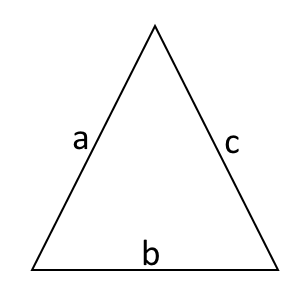#### All fields are required:

a
b
c
Area =
0
Perimeter =
0
ab Angle =
0 deg
ac Angle =
0 deg
bc Angle =
0 deg
*For decimal dimensions, use the dot notation! (.)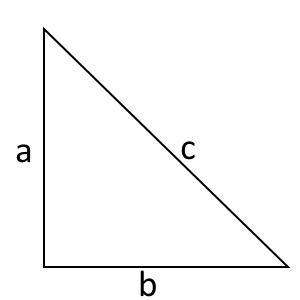#### Only 2 dimensions (any) are required:

a
b
c
Area =
0
Perimeter =
0
a =
0
b =
0
c =
0
ab Angle =
90 deg
ac Angle =
0 deg
bc Angle =
0 deg
*For decimal dimensions, use the dot notation! (.)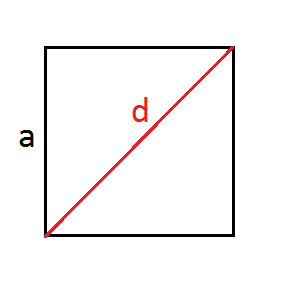#### Only one field is required (any):

a
d
Area =
0
Perimeter =
0
a =
0
d =
0
45 deg
*For decimal dimensions, use the dot notation! (.)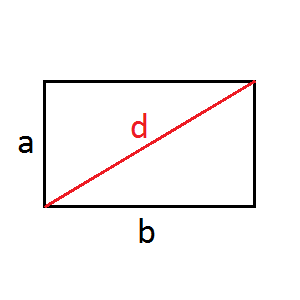#### Please fill ANY 2 dimensions:

a
b
d
Area =
0
Perimeter =
0
a =
0
b =
0
d =
0
0 deg
db Angle =
0 deg
*For decimal dimensions, use the dot notation! (.)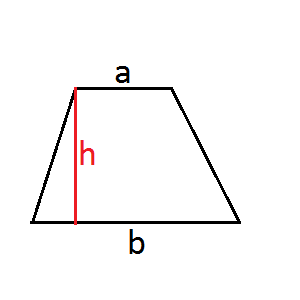#### All fields are required:

a
b
d
Area
0
Perimeter
0
*For decimal dimensions, use the dot notation! (.)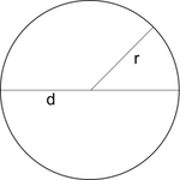r
Circumference:
0
Area:
0
Diameter:
0
*For decimal dimensions, use the dot notation! (.)